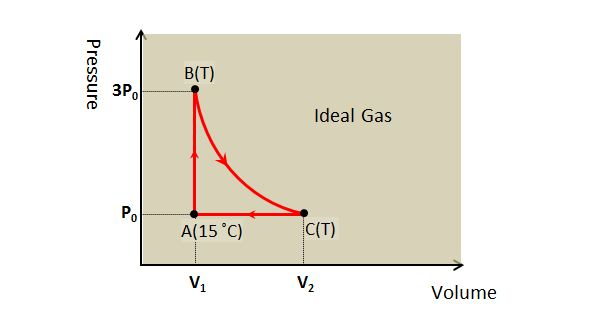# Circulation of Ideal gasThe above diagram shows circulation of an ideal gas by the sequence A $\to$ B $\to$ C $\to$A. The temperature of the ideal gas at point A is $15$ °C, and the temperature from B to C remains constant at T.

Which of the following is correct?

a) T=$45$ °C.
b) V$_2$=$3$V$_1$.
c) The process A $\to$ B is related to Boyle's law.

×